# PSI Variables by Alpha¶

Note

Lowercase letters in PSI variable names represent portions of the variable name that vary by root number, calculation order, etc. See text for fuller description.

(T) CORRECTION ENERGY

The coupled-cluster perturbative triples correction [H].

AAA (T) CORRECTION ENERGY
AAB (T) CORRECTION ENERGY
ABB (T) CORRECTION ENERGY
BBB (T) CORRECTION ENERGY

Components of the coupled-cluster perturbative triples correction [H].

BRUECKNER CONVERGED

Value 1 (0) when the Brueckner orbitals have (have not) converged.

CBS TOTAL ENERGY
CBS CORRELATION ENERGY
CBS REFERENCE ENERGY

The total electronic energy [H] and its breakdown into reference total energy [H] and correlation correction components [H] for the compound method requested through cbs().

CC ROOT n TOTAL ENERGY

The total electronic energy [H] for the requested coupled cluster level of theory and root n (numbering starts at GS = 0).

CC TOTAL ENERGY
CC CORRELATION ENERGY
CC2 TOTAL ENERGY
CC2 CORRELATION ENERGY

The total electronic energy [H] and correlation energy component [H] for the CC2 level of theory.

CC3 TOTAL ENERGY
CC3 CORRELATION ENERGY

The total electronic energy [H] and correlation energy component [H] for the CC3 level of theory.

CCSD TOTAL ENERGY
CCSD CORRELATION ENERGY

The total electronic energy [H] and correlation energy component [H] for the CCSD level of theory.

CCSD(T) TOTAL ENERGY
CCSD(T) CORRELATION ENERGY

The total electronic energy [H] and correlation energy component [H] for the CCSD(T) level of theory.

CI DIPOLE X
CI DIPOLE Y
CI DIPOLE Z

The three components of the dipole [Debye] for the requested configuration interaction level of theory and root.

The six components of the quadrupole [Debye Ang] for the requested configuration interaction level of theory and root.

CI ROOT n -> ROOT m DIPOLE X
CI ROOT n -> ROOT m DIPOLE Y
CI ROOT n -> ROOT m DIPOLE Z

The three components of the transition dipole [Debye] between roots n and m for the requested configuration interaction level of theory.

CI ROOT n -> ROOT m QUADRUPOLE XX
CI ROOT n -> ROOT m QUADRUPOLE XY
CI ROOT n -> ROOT m QUADRUPOLE XZ
CI ROOT n -> ROOT m QUADRUPOLE YY
CI ROOT n -> ROOT m QUADRUPOLE YZ
CI ROOT n -> ROOT m QUADRUPOLE ZZ

The three components of the transition quadrupole [Debye Ang] between roots n and m for the requested configuration interaction level of theory.

CI ROOT n DIPOLE X
CI ROOT n DIPOLE Y
CI ROOT n DIPOLE Z

The three components of the dipole [Debye] for the requested configuration interaction level of theory and root n.

The six components of the quadrupole [Debye Ang] for the requested configuration interaction level of theory and root n.

CI ROOT n TOTAL ENERGY
CI ROOT n CORRELATION ENERGY

The total electronic energy [H] and correlation energy component [H] for the requested configuration interaction level of theory and root n (numbering starts at 1).

CI STATE-AVERAGED TOTAL ENERGY
CI STATE-AVERAGED CORRELATION ENERGY

The total electronic energy [H] and correlation energy component [H] for state-averaged CI/CASSCF levels of theory.

CI TOTAL ENERGY
CI CORRELATION ENERGY

The total electronic energy [H] and correlation energy component [H] for the requested configuration interaction level of theory and root.

CISD TOTAL ENERGY
CISD CORRELATION ENERGY
CISDT TOTAL ENERGY
CISDT CORRELATION ENERGY
CISDTQ CORRELATION ENERGY
CISDTQ TOTAL ENERGY
CIn CORRELATION ENERGY
CIn TOTAL ENERGY

The total electronic energy [H] and correlation energy component [H] for the labeled configuration interaction level of theory and root. n is CI order for n > 4.

CP-CORRECTED 2-BODY INTERACTION ENERGY

The interaction energy [H] considering only two-body interactions, computed with counterpoise correction. Related variable UNCP-CORRECTED 2-BODY INTERACTION ENERGY.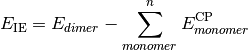CURRENT CORRELATION ENERGY

The correlation energy [H] corresponding to the CURRENT ENERGY variable.

CURRENT ENERGY

The total electronic energy [H] of the most recent stage of a calculation (frequently overwritten). This is the quantity tracked by the geometry optimizer.

CURRENT REFERENCE ENERGY

The total electronic energy [H] of the reference stage corresponding to the CURRENT ENERGY variable.

db_name DATABASE MEAN ABSOLUTE DEVIATION

The mean absolute deviation [kcal mol-1] of the requested method name from the stored reference values for the requested reactions in database db_name. If no reference is available, this will be a large and nonsensical value.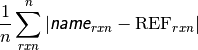db_name DATABASE MEAN SIGNED DEVIATION

The mean deviation [kcal mol-1] of the requested method name from the stored reference values for the requested reactions in database db_name. If no reference is available, this will be a large and nonsensical value.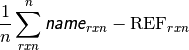db_name DATABASE ROOT-MEAN-SQUARE SIGNED DEVIATION

The rms deviation [kcal mol-1] of the requested method name from the stored reference values for the requested reactions in database db_name. If no reference is available, this will be a large and nonsensical value.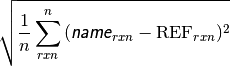DF-MP2 TOTAL ENERGY
DF-MP2 CORRELATION ENERGY

The total electronic energy [H] and correlation energy component [H] for the density-fitted MP2 level of theory.

DFT FUNCTIONAL ENERGY

The functional energy contribution [H] to the total SCF energy (DFT only). Quantity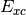in Eq. (2).

DFT FUNCTIONAL TOTAL ENERGY

The total electronic energy [H] for the underlying functional of the requested DFT method, without any dispersion correction, the first four terms in Eq. (2). When the requested method includes a dispersion correction, this quantity is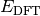in Eq. (1). Otherwise, quantity equal to DFT TOTAL ENERGY and SCF TOTAL ENERGY.

DFT TOTAL ENERGY

The total electronic energy [H] for the requested DFT method,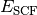in Eq. (2). When the method includes a dispersion correction, this quantity is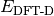in Eq. (1).

DISPERSION CORRECTION ENERGY

The dispersion correction [H] appended to an underlying functional when a DFT-D method is requested. Quantity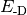in Eqs. (1) and (2).

(1)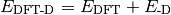FCI TOTAL ENERGY
FCI CORRELATION ENERGY

The total electronic energy [H] and correlation energy component [H] for the full configuration interaction level of theory.

LCC2 (+LMP2) TOTAL ENERGY

The total electronic energy [H] for the local CC2 level of theory.

LCCSD (+LMP2) TOTAL ENERGY

The total electronic energy [H] for the local CCSD level of theory.

MP2 TOTAL ENERGY
MP2 CORRELATION ENERGY

The total electronic energy [H] and correlation energy component [H] for the MP2 level of theory.

MP2.5 TOTAL ENERGY
MP2.5 CORRELATION ENERGY

The total electronic energy [H] and correlation energy component [H] for the MP2.5 level of theory.

MP3 TOTAL ENERGY
MP3 CORRELATION ENERGY

The total electronic energy [H] and correlation energy component [H] for the MP3 level of theory.

MPn TOTAL ENERGY
MPn CORRELATION ENERGY

The total electronic energy [H] and correlation energy component [H] for the labeled Möller–Plesset perturbation theory level. n is MP perturbation order.

NUCLEAR REPULSION ENERGY

The nuclear repulsion energy contribution [H] to the total SCF energy. Quantity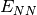in Eq. (2).

ONE-ELECTRON ENERGY

The one-electron energy contribution [H] to the total SCF energy. Quantity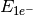in Eq. (2).

SAPT DISP ENERGY
SAPT ELST ENERGY
SAPT EXCH ENERGY
SAPT IND ENERGY

Respectively, the dispersion, electrostatics, exchange, and induction components of the total electronic interaction energy [H] for the the requested SAPT level of theory. The sum of these four components yields SAPT ENERGY.

SAPT ENERGY

The total electronic interaction energy [H] for the requested SAPT level of theory.

SAPT SAPT0 ENERGY
SAPT SAPT2 ENERGY
SAPT SAPT2+ ENERGY
SAPT SAPT2+(3) ENERGY
SAPT SAPT2+3 ENERGY

The total electronic interaction energy [H] for the labeled SAPT level of theory.

SCF TOTAL ENERGY

The total electronic energy [H] of the SCF stage of the calculation. The CORRELATION ENERGY variables from subsequent stages of a calculation are often the corresponding TOTAL ENERGY variables less this quantity. Constructed from Eq. (2).

(2)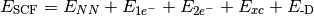TWO-ELECTRON ENERGY

The two-electron energy contribution [H] to the total SCF energy. Quantity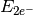in Eq. (2).

UNCP-CORRECTED 2-BODY INTERACTION ENERGY

The interaction energy [H] considering only two-body interactions, computed without counterpoise correction. Related variable CP-CORRECTED 2-BODY INTERACTION ENERGY.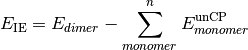ZAPTn TOTAL ENERGY
ZAPTn CORRELATION ENERGY

The total electronic energy [H] and correlation energy component [H] for the labeled Z-averaged perturbation theory level. n is ZAPT perturbation order.

#### Previous topic

Keywords by Alpha

#### Next topic

Keywords by Module